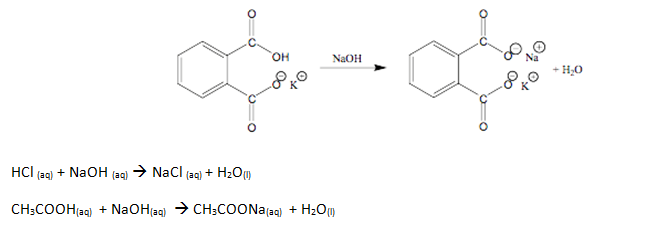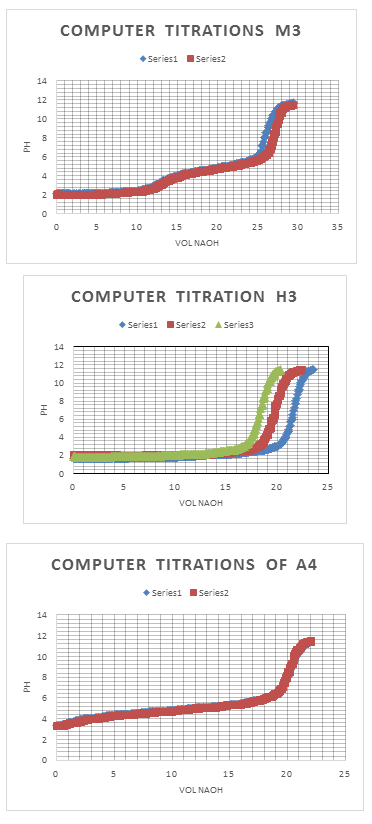# Preparing Standard Acid and Base – Finding Molarity

Preparing Standard Acid and Base & Finding Molarity
By: Arturo Morillo

Introduction:

The purpose of this laboratory is to learn how to prepare the standard acid and base that will be used in the laboratory. These acid and bases will be used throughout the laboratory. By standardizing them, we are able to calculate their exact concentrations. We will be using potassium hydrogen phthalate to determine the concentration of sodium hydroxide. Four trials will be taken to to calculate the concentration of sodium hydroxide. This concentration will later be used to titrate three unknowns, unknown H3, A4, and M3.Experimental Procedures:

The experiment began by standardizing sodium hydroxide (KHP). To do this, an amount of potassium hydrogen phthalate wad dried for an hour and placed in a desiccators. Then, 500 mL of distilled water was placed in a polyethylene bottle with 2.8 mL of 50 wt% NaOH and the mixture was mix for a few minutes. Four samples of 0.510 g of solid KHP were weighed and dissolved in 25 mL of distilled water in a 125 mL flask. After the KHP was dissolved in each sample, 3 drops of phenolphthalein were added to each flask. A 50 mL buret was filled with standardized NaOH and used to titrate each flask. The endpoint was noted each time a flask was titrated by the change in color of the solution. Four titration trials were taken and used to find the molarity of NaOH. Using the NaOH, three unknowns were titrate. These unknowns were H3, A4, and M3. In order to titrate these unknowns, 2.5 mL of each unknown was placed in separate 125 mL flasks. Same as the procedure before, 3 drops of phenolphthalein were added to each flask, to be titrated with NaOH. After the titrations were all complete, a computerized Verneir system was used to calculate the volume used to reach the endpoint of each unknown. The procedure of the titrations was similar with the procedures before, the only difference was that the system was more accurate and more precise.

Results and Discussion:

Results:

The results of the experiment were really conclusive. The trials all were within a small standard deviation and seemed to match for the most part. Looking at the data, the molarities of the unknowns done by hand were off from the molarity that was gotten by the computerized Vernier system. The only error, that be seen to cause the discrepancy between the data, might have been the point in which the solution changes color due to the indicator being used. Since the Vernier system is a computerized calculation, there is a smaller room for error, whereas when using an indicator there can be many sources of errors. One of these errors can be seen in the different coloration of the indicator. This means that some people might find the endpoint to be different depending on what they feel the correct color is.

Data:

“Standardization of NaOH”

 Trial # Mass (g) Mole (mol) Molarity (M) 1 0.5135 0.00 33.07 33.07 0.002514 0.0760 2 0.5103 0.00 32.85 32.85 0.002499 0.0761 3 0.5118 0.00 33.00 33.00 00.002506 0.0759 4 0.5175 0.00 33.40 33.40 00.002534 0.0759

x̅ = 0.07597 M or 0.264% NaOH

σ = 9.57 x 10-5 M

% RSD = 0.126%

Confidence Interval = 0.07597 +/- 9.57 x 10-5 M

“Titration of Unknown M3 (Mixture)”

 Trial Amount of Unknown (mL) Molarity (M) 1 2.50 0.00 26.55 26.55 0.8068 2 2.50 4.60 30.78 26.18 0.7955 3 2.50 8.80 35.05 26.25 0.7977

x̅ = 0.800 M M3

σ = 0.006 M

% RSD = 0.75%

Confidence Interval = 0.800 +/- 0.006 M

“Titration of Unknown H3 (Hydrochloric Acid)”

 Trial Amount of Unknown (mL) Molarity (M) 1 2.50 10.70 30.38 19.68 0.5980 2 2.50 2.35 21.90 19.55 0.5941 3 2.50 7.90 26.65 18.75 0.5698

x̅ = 0.5873 M H3

σ = 0.015 M

% RSD = 2.55%

Confidence Interval = 0.5873 +/- 0.015 M

“Titration of Unknown A4 (Acetic Acid)”

 Trial Amount of Unknown (mL) Molarity (M) 1 2.50 1.93 26.38 24.45 0.7430 2 2.50 0.00 24.55 24.55 0.7460 3 2.50 24.55 44.68 24.13 0.7333

x̅ = 0.7408 M A4

σ = 0.0066 M

% RSD = 0.89%

Confidence Interval = 0.741 +/- 0.0066 M

1. “Computer titration of Unknown H3”
 Trial Amount of Unknown (mL) pH Molarity (M) 1 2.50 21.596 7.012 0.6562 2 2.50 18.35 7.170 0.5576 3 2.50 19.717 7.069 0.5992

x̅ = 0.6043 M H3

σ = 0.049 M

% RSD = 8.12%

Confidence Interval = 0.60 +/- 0.049 M

1. “Computer titration of Unknown “A4”
 Trial Amount of Unknown (mL) pH Molarity (M) 1 2.50 20.280 8.783 0.6163 2 2.50 20.156 8.861 0.6125

x̅ = 0.6144 M A4

σ = 0.00268 M

% RSD = 0.436%

Confidence Interval = 0.614 +/- 0.00268 M

1. “Computer titration of Unknown M3”
 Trial Amount of Unknown (mL) pH pH Molarity (M) 1 2.50 13.000 3.167 26.126 8.686 0.7939 2 2.50 12.692 3.210 26.114 8.542 0.7936

x̅ = 0.7937 M M3

σ = 0.000212 M

% RSD = 0.027%

Confidence Interval = 0.7937 +/- 0.000212 M

Calculations of Data:

Sample Calculations (Using Trial One of Standardization of NaOH)

0.5135g KHP   x    1 mol KHP     = 0.002514 mol KHP

1                   204.22g KHP

0.002514 mol KHP   x    1 mol NaOH     = 0.002514 mol NaOH

1                            1 mol KHP

0.002514 mol NaOH     = 0.0760 M NaOH

0.03307 L

Sample Calculations (Using Trial One of Standardization of H3)

0.07597 M NaOH   x    26.55 mL NaOH     = 0.0020117 mol NaOH

1                                              1000 mL

0.0020117 mol NaOH =        0.002017 mol HCl            = 0.8068 M HCl

2.50 mL unknown/1000 mL

Same calculations were used for the Computer Titrations Calculations as in for H3

Discussion:

In conclusion, the experiment was a success. There was a small difference from the titrations completed with an indicator and the titrations completed with the computerized Vernier system. As stated in the results, this difference can be attributed to human error. While the Vernier system calculates the pH drop by drop, the error in determining the endpoint with an indicator is great. Depending on the person, the solution could reach endpoint on different color shades, since not everyone sees the same color. Overall, the standard deviation for most of the data was rather small. This experiment does reinstate the fact that the Vernier system is more reliable than using an indicator for titrating unknown solutions.• Share